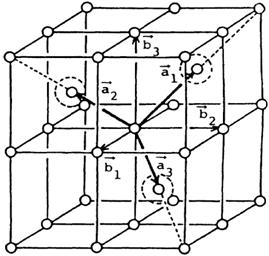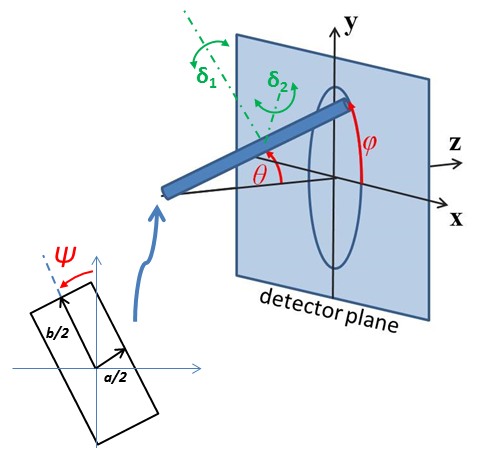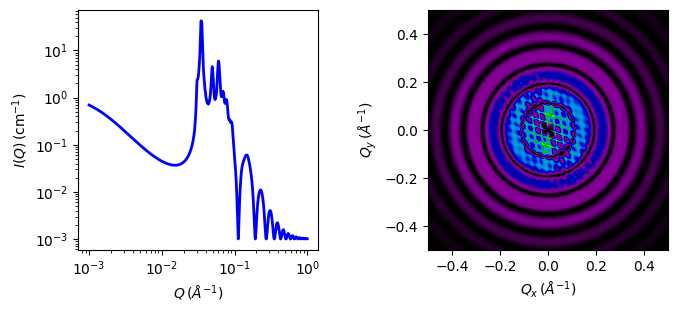# bcc_paracrystal

Body-centred cubic lattic with paracrystalline distortion

Parameter Description Units Default value
scale Scale factor or Volume fraction None 1
background Source background cm-1 0.001
dnn Nearest neighbour distance 220
d_factor Paracrystal distortion factor None 0.06
sld Particle scattering length density 10-6-2 4
sld_solvent Solvent scattering length density 10-6-2 1
theta c axis to beam angle degree 60
phi rotation about beam degree 60
psi rotation about c axis degree 60

The returned value is scaled to units of cm-1 sr-1, absolute scale.

Warning

This model and this model description are under review following concerns raised by SasView users. If you need to use this model, please email help@sasview.org for the latest situation. The SasView Developers. September 2018.

Definition

Calculates the scattering from a body-centered cubic lattice with paracrystalline distortion. Thermal vibrations are considered to be negligible, and the size of the paracrystal is infinitely large. Paracrystalline distortion is assumed to be isotropic and characterized by a Gaussian distribution.

The scattering intensity $$I(q)$$ is calculated as

$I(q) = \frac{\text{scale}}{V_p} V_\text{lattice} P(q) Z(q)$

where scale is the volume fraction of spheres, $$V_p$$ is the volume of the primary particle, $$V_\text{lattice}$$ is a volume correction for the crystal structure, $$P(q)$$ is the form factor of the sphere (normalized), and $$Z(q)$$ is the paracrystalline structure factor for a body-centered cubic structure.

Equation (1) of the 1990 reference is used to calculate $$Z(q)$$, using equations (29)-(31) from the 1987 paper for $$Z1$$, $$Z2$$, and $$Z3$$.

The lattice correction (the occupied volume of the lattice) for a body-centered cubic structure of particles of radius $$R$$ and nearest neighbor separation $$D$$ is

$V_\text{lattice} = \frac{16\pi}{3} \frac{R^3}{\left(D\sqrt{2}\right)^3}$

The distortion factor (one standard deviation) of the paracrystal is included in the calculation of $$Z(q)$$

$\Delta a = g D$

where $$g$$ is a fractional distortion based on the nearest neighbor distance.Fig. 51 Body-centered cubic lattice.

For a crystal, diffraction peaks appear at reduced q-values given by

$\frac{qD}{2\pi} = \sqrt{h^2 + k^2 + l^2}$

where for a body-centered cubic lattice, only reflections where $$(h + k + l) = \text{even}$$ are allowed and reflections where $$(h + k + l) = \text{odd}$$ are forbidden. Thus the peak positions correspond to (just the first 5)

$\begin{split}\begin{array}{lccccc} q/q_o & 1 & \sqrt{2} & \sqrt{3} & \sqrt{4} & \sqrt{5} \\ \text{Indices} & (110) & (200) & (211) & (220) & (310) \\ \end{array}\end{split}$

Note

The calculation of $$Z(q)$$ is a double numerical integral that must be carried out with a high density of points to properly capture the sharp peaks of the paracrystalline scattering. So be warned that the calculation is slow. Fitting of any experimental data must be resolution smeared for any meaningful fit. This makes a triple integral which may be very slow.

This example dataset is produced using 200 data points, qmin = 0.001 Å-1, qmax = 0.1 Å-1 and the above default values.

The 2D (Anisotropic model) is based on the reference below where $$I(q)$$ is approximated for 1d scattering. Thus the scattering pattern for 2D may not be accurate, particularly at low $$q$$. For general details of the calculation and angular dispersions for oriented particles see Oriented particles. Note that we are not responsible for any incorrectness of the 2D model computation.Fig. 52 Orientation of the crystal with respect to the scattering plane, when $$\theta = \phi = 0$$ the $$c$$ axis is along the beam direction (the $$z$$ axis).Fig. 53 1D and 2D plots corresponding to the default parameters of the model.

Source

bcc_paracrystal.py $$\ \star\$$ bcc_paracrystal.c $$\ \star\$$ sphere_form.c $$\ \star\$$ gauss150.c $$\ \star\$$ sas_3j1x_x.c

References

  Hideki Matsuoka et. al. Physical Review B, 36 (1987) 1754-1765 (Original Paper)
  Hideki Matsuoka et. al. Physical Review B, 41 (1990) 3854-3856 (Corrections to FCC and BCC lattice structure calculation)

Authorship and Verification

• Author: NIST IGOR/DANSE Date: pre 2010